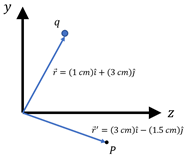# Problem: A 6 μC charge moves with a velocity of. v = (1.2 km/s) î  - (2.4 km/s) ĵ. What is the magnetic field, in vector form, at the point P indicated in the following figure?

###### FREE Expert Solution
100% (268 ratings)
###### Problem Details

A 6 μC charge moves with a velocity of. v = (1.2 km/s) î  - (2.4 km/s) ĵ. What is the magnetic field, in vector form, at the point P indicated in the following figure?